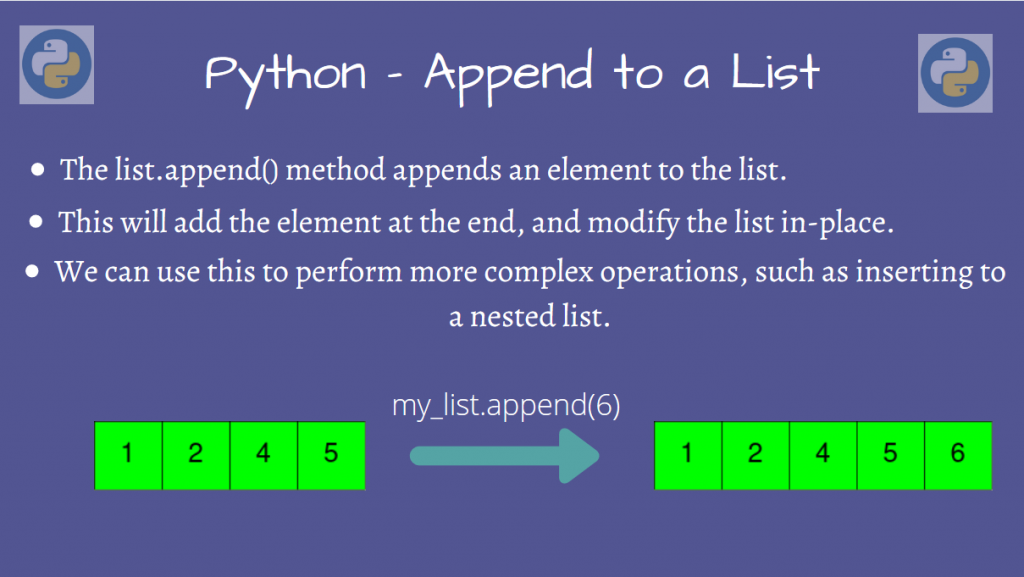# Append to a List in PythonIn this article, we’ll take a look at how we can append to a List in Python.

Python’s list.append() provides the solution to this, so we’ll see some examples using this method.

Let’s get started!

## Append to a normal List in Python

We can use Python’s built-in append() method on our List, and add our element to the end of the list.

```my_list = [2, 4, 6, 8]

print("List before appending:", my_list

# We can append an integer
my_list.append(10)

# Or even other types, such as a string!
my_list.append("Hello!")

print("List after appending:", my_list)
```

Output

```List before appending: [2, 4, 6, 8]
List after appending: [2, 4, 6, 8, 10, "Hello!"]
```

As you can observe, our list has the two elements 10 and “Hello” inserted at the end. This is the case when you’re appending to a normal list.

Let’s now look at some other cases now.

## Append to a List in Python – Nested Lists

A Nested List is a List that contains another list(s) inside it. In this scenario, we will find out how we can append to a list in Python when the lists are nested.

We’ll look at a particular case when the nested list has N lists of different lengths. We want to insert another list of exactly N elements into our original list.

But now, instead of directly appending to the nested list, we’ll be appending each of the N elements to each of the N lists, in order.

To show you an example, here’s our nested list having N = 3 lists:

```nested_list = [[1, 2, 3], [4, 5, 6, 7], [2, 4, 5, 6, 7]]
```

We’ll insert each of the N elements of the list:

```my_list = [10, 11, 12]
```

10 will be appended to the first list, 11 to the second, and 12 to the third.

So, our output will be:

```[[1, 2, 3, 10], [4, 5, 6, 7, 11], [2, 4, 5, 6, 7, 12]]
```

Got the problem? Let’s solve it now!

So, for each list in our nested list, we pick the corresponding element from `my_list` and append it to that list. We keep doing this until we reach the end of the nested list, as well as `my_list`.

A possible approach would be to iterate through the nested list. Since we know that every element of the nested list is a list, we can take the index of the current element, and append `my_list[idx]` to `nested_list[idx]`.

```nested_list = [[1, 2, 3], [4, 5, 6, 7], [2, 4, 5, 6, 7]]

my_list = [10, 11, 12]

for idx, small_list in enumerate(nested_list):
small_list.append(my_list[idx])

print(nested_list)
```

Output

```[[1, 2, 3, 10], [4, 5, 6, 7, 11], [2, 4, 5, 6, 7, 12]]
```

Indeed, our output matches what we expected!

## Conclusion

In this article, we learned how we could append to a Python List, and examined various cases for this process.